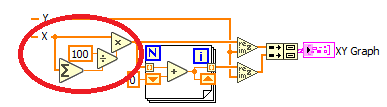# LabVIEW

cancel
Showing results for
Did you mean:

Solved!
Go to solution

## Re: Plot a graph with the value distribution in percent

Histogram data I have already. The problem was, I was not sure, what I was doing, so It is difficult to explain what I wanted ‌‌.

I got frequency data, I calculate the rams values for 5 mins in 5 sec interval (60 values). And I want to make a diagram as exactly the one you have posted, similar to mine that I have posted. My uni supervisor has no idea and the company I am writing my thesis doesn’t have time to explain. So, I am just trying to figure out by reading the norm and googling form the internet. So far most of the stuffs are clear, but now I am trying to get the number 2, calculating the integral of the histogram.

And about your last paragraph questions. It seemed Hebrew at first, but now I got some idea‌‌. My data are so massed that it will make no sense if I upload.

One last question. The integral of the histogram: to convert it into percent, do I have to divide with max value or summation. Because in the picture that you have provided, it doesn't look like divided by summation to get percentage. And thank you a lot again for rescuing me.

Message 11 of 12
(112 Views)
Solution
Accepted by topic author mub.re

## Re: Plot a graph with the value distribution in percent

@mub.re wrote:

One last question. The integral of the histogram: to convert it into percent, do I have to divide with max value or summation. Because in the picture that you have provided, it doesn't look like divided by summation to get percentage. And thank you a lot again for rescuing me.

You don't get percent by dividing by the sum. You also need to multiply by 100. Is is easier to divide 100 by the sum and multiply that with all the data as I show in the picture. Now both are "normalized to percentages and the sum will add up to 100%)(Dividing by the sum is the same as dividing by the max value of the integral, but since we normalize first, the running sum will automatically go to 100. The order of operations does not matter but if you calculate the sum first, you need to later normalize twice as much data (the data AND the integral) so keep it simple, as I did!)LabVIEW Champion.Message 12 of 12
(106 Views)